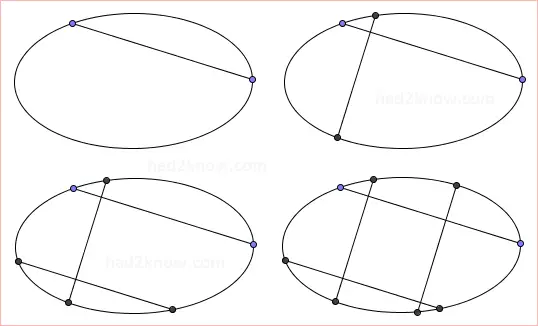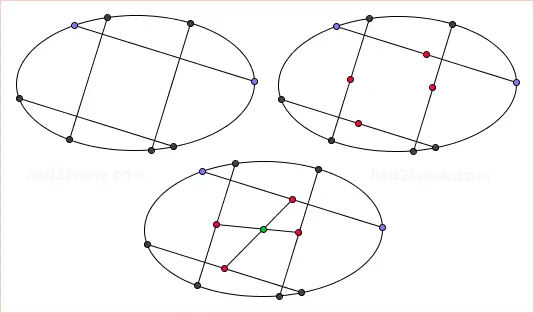# How to Find the Exact Center of an Ellipse

An ellipse is one type of conic section curve; it is closed curve similar to a circle except that it is longer in one direction and shorter in the perpendicular direction.

If you are given an ellipse with no markings and you don't know its dimensions, you can still locate its exact geometric center. You will need a straight edge, ruler, right angle, and either a pencil or string and tape to demarcate the chord lines.

Step 1: Draw a chord somewhere across the ellipse.

Step 2: Draw a second chord perpendicular to the first so that the two chords intersect somewhere inside the ellipse. Ideally, they should intersect close to the border of the ellipse.

Step 3: Draw a third chord perpendicular to the second (and parallel to the first), again making sure that the two intersect within the ellipse.

Step 4: Finally, draw a fourth chord perpendicular to the third (and parallel to the second) so that they intersect within the ellipse. See figure below for an illustration of Steps 1 through 4:After completing Steps 1 through 4 you should have four line segments (two pairs of two parallel lines) that form a rectangle inside the ellipse.

Step 5: Use the ruler to find the midpoints of each of the four lines.

Step 6: Draw two line segments, each connecting a midpoint to its midpoint friend on the parallel line. Where these segments intersect is the center of the ellipse.Once you know where the center of an ellipse is, you can then find the major axis, minor axis, and two foci. See companion article for finding the axes and foci of an ellipse.